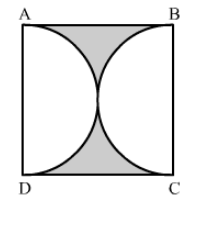# In the given figure, find the area of the shaded region,`
Question:

In the given figure, find the area of the shaded region, if ABCD is a square of side 14 cm and APD and BPC are
semicircles.Solution:

Area of the shaded region = Area of Square ABCD − (Area of semicircle APD + Area of semicircle BPC)

$=(14)^{2}-\left[\frac{1}{2} \pi\left(\frac{14}{2}\right)^{2}+\frac{1}{2} \pi\left(\frac{14}{2}\right)^{2}\right]$

$=(14)^{2}-\frac{22}{7}(7)^{2}$

$=42 \mathrm{~cm}^{2}$

Hence, the area of the shaded region is 42 cm2.